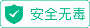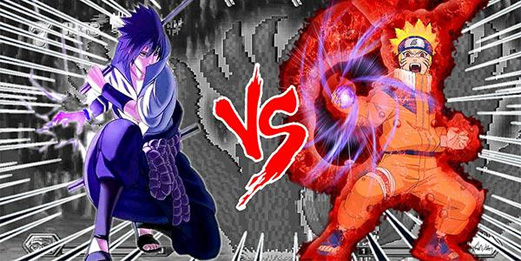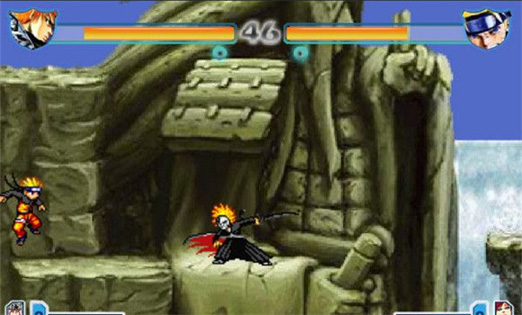# 死神VS火影  1.5• 软件授权： 免费软件
• 软件大小： 7.01MB
• 软件评分：
• 软件类型： 国产软件
• 更新时间： 2021-12-13
• 应用平台： Winxp/vista/win7/win8/2000/2003
• 软件语言： 简体中文
• 版       本： 1.5玩家：2←→左右移动;↓防御;1攻击;2跳跃;3冲刺;4远攻;5必杀;6召唤援助;

如何开始：游戏加载完毕用WSAD键选择模式再用J键确定 - 接着按J键 - 之后选择角色 - 然后选择援助即可开始游戏

游戏目标：合理操作控制角色战胜自己的对手吧!1、黑崎一护 J+K 卍解

普通状态：J 砍一下 J+J 二连击 J+J+J 三连击 K 跳一下 K+K 大跳 K+J 跳起来砍一下 W+J 向上的旋风 S+J 向前震一下 W+U 向斜上的波 S+U 向下的波 I 小招(费一格气) W+I 向上的小招(费一格气) S+I 大招(费三格气)

卍解状态：J 砍一下 J+J 二连击 J+J+J 四连击 K 跳一下 K+K 大跳 K+J 跳起来砍一下 W+J 向上冲起来砍一下 S+J 向前震一下 W+J 向斜上砍 S+U 向下的波 I 小招(费一格气) W+I 连砍(费一格气) S+I 大招(费三格气) PS：速度会变快。2、漩涡鸣人： J+K 九尾化

普通状态

J 打一拳 J+J 二连击 J+J+J 三连击 K 跳一下 K+K 大跳 K+J 跳起来打一拳 W+J 分一个影分身揍一顿 S+J螺旋丸 W+U 向斜上打一下 S+U 风魔手里剑 I 大玉螺旋丸(费一格气) W+I 分一群影分身揍一顿(费一格气) S+I 鸣人连弹+螺旋丸(费三格气) 。

九尾状态

J 抓一下 J+J 二连击 J+J+J 三连击 J+J+J+J 四连击 K 跳一下 K+K 大跳 K+J 跳起来踢一下 W+J 九尾手抓过来打一拳 S+J 螺旋丸 W+U 旋风 S+U 暴气 I 螺旋丸(牛一些)(费一格气) W+I 变四尾把敌人打上去(费一格气) S+I 大波(费三格气) PS：会费血。3、朽木露琪亚

J 踢一下 J+J 二连击 J+J+J 三连击 K 跳一下 K+K 大跳 K+J 跳起来踢一下 W+J 向斜上踢 S+J 闪到身后打飞敌人 W+U 向斜上的波 S+U 暴一下? I 小招(费一格气) W+I 同上(费一格气) S+I 变死神(费三格气)。4、春野樱

J 打一下 J+J 二连击 J+J+J 三连击 K 跳一下 K+K 大跳 K+J 跳起来踢一下 W+J 倒立+踢 S+J 扔石头 W+U 向斜上的苦无 S+U 向下打一拳 I 小招(费一格气) W+I 同上(费一格气) S+I 小樱的内心(费三格气)。5、日番谷冬狮郎

J 砍一下 J+J 二连击 J+J+J 三连击 K 跳一下 K+K 大跳 K+J 跳起突袭 W+J 突袭 S+J 暴气 W+U 向斜上砍 S+U 向外暴气 I 冰龙(费一格气) W+I 冰柱(费一格气) S+I 大招(费三格气)。## 网友评论华军网友华军网友华军网友

#### 本周最热软件本周最新软件